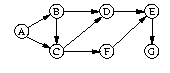COSC-270: Artificial Intelligence

Project 1
Fall 2017

Due: R 9/28 @ 5 PM
4 points

1. Write the function min-max-i that uses the do form to find the minimum and maximum values in a list of numbers. The function should return these two values in a list. Do not use the min and max functions in your implementation.

2. Same problem as above, but use recursion to implement the function min-max-r.

3. Same problem as above, but use apply, min, and max to implement min-max-a.

4. Encode the following tree as a list assigned to a globally declared variable. Write two Lisp functions, preorder and postorder, that return as a list the pre-order and post-order traversals of the tree passed in as the argument, respectively.For example,

> (preorder *tree*)
(O R E G O ... )

5. Encode the following graph using property lists. Write the function dfs that, when given a start and an end node, uses depth-first search to return a path between them.For example,

>(dfs 'a 'g)
(A C F E G)

>(dfs 'f 'g)
(F E G)

>(dfs 'e 'c)
NIL

6. Using a 3x3 array to represent a tic-tac-toe board, write the successors function that, when given a board configuration and a player's token as arguments, returns a list containing all of the next possible boards. Use nil to represent open positions. Use o and x to represent occupied positions.

7. Tanimoto defined a heuristic evaluation function for tic-tac-toe boards as

f = 100A + 10B + C - (100D + 10E + F),

where

• A is the number of lines with 3 X's,
• B is the number of unblocked lines w/ 2 X's,
• C is the number of unblocked lines with 1 X,
• D is the number of lines with 3 O's,
• E is the number of unblocked lines w/ 2 O's, and
• F is the number of unblocked lines w/ 1 O.

Implement Tanimoto's heuristic evaluation function as the Lisp function tanimoto. That is, when given a tic-tac-toe board represented as a 3x3 array, the function returns f.

Instructions for Electronic Submission

The name of the file containing your Lisp functions must be named main.lisp. In a file named HONOR, provide the following information:
Name
NetID

In accordance with the class policies and Georgetown's Honor Code,
I certify that, with the exceptions of the course materials and those
items noted below, I have neither given nor received any assistance
on this project.